1. The current sensitivity of a moving coil galvanometer increases with decrease in :

(A) magnetic field
(B) area of a coil
(C) number of turns
(D) None of these

2. Acurrent carrying coil is placed in a uniform magnetic field. If the coll turns through an angle O, then the torque is directly proportional to :

(A) sin θ
(B) cos θ
(C) cot θ
(D) tan θ

3. The sensitivity of a tangent galvanometer can be increased by increasing :

(A) the radius of the coil
(B) the external magnetic field
(C) the number of turns of the coil
(D) all of the above

4. The permeability of a paramagnetic substance is :

(A) very large
(B) small but more than unity
(C) less than unity
(D) negative

5. Which of the following shows that the earth behaves as a magnet ?

(A) Repulsion between like poles
(B) Attraction between unlike poles
(C) Null points in the magnetic field of a bar magnet
(D) No existence of isolated magnetic poles

6. What is the angle of dip at the magnetic poles ?

(A) 30°
(B) 0°
(C) 45°
(D) None of these

7. A charged particle of mass m and charge a travels on a circular path of radius r i.e., perpendicular to the magnetic field B. The time taken by particle to complete on revoiution is :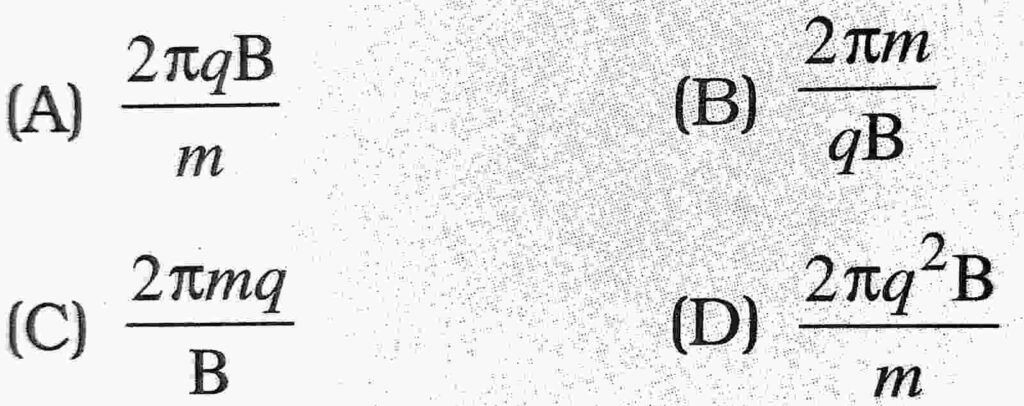8. Circular loop of radius 0.0157 m carries a current 2A. The magnetic field at the centre of the loop is :

(A) 1.57 x 10-3 Wb/m2
(B) 8.0 x 10-5 Wb/m2
(C) 2.0 x 10-3 Wb/m2
(D) 3.14 x 10-1 Wb/m2

9. What happens to the magnetic field at the centre of a circular current carrying coil if we double the radius of the coil keeping the current unchanged ?

(A) halved
(B) doubled
(D) remains unchanged

10. When we double the radius of a coil keeping the current through it unchanged, what happens to the magnetic field directed along its axis at far off points ?

(A) halved
(B) doubled
(D) remains unchanged

11. The strength of the magnetic field around an infinite current carrying conductor is

(A) same everywhere
(B) inversely proportional to the distance
(C) directly proportional to the distance
(D) None of these

12. A current carrying power line carries current from west to east. Then the direction of the magnetic field 2 m above it is :

(A) west to east
(B) south to north
(C) north to south
(D) None of these

13. According to Ampere’s Circuital law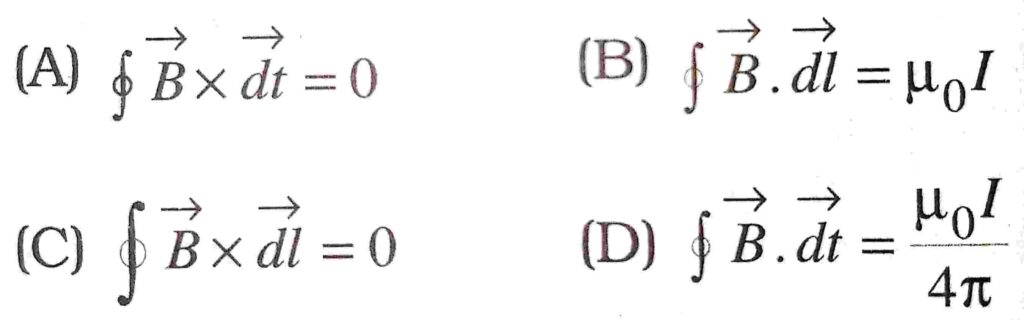14. The force between two parallel current carrying conductors is F. If the current in each conductor is doubled, then the force between them becomes :

(A) 4F
(B) 2F
(C) F
(D) F/4

15. How much force will be experienced by a moving charge in a magnetic field ? The symbols have their usual meanings :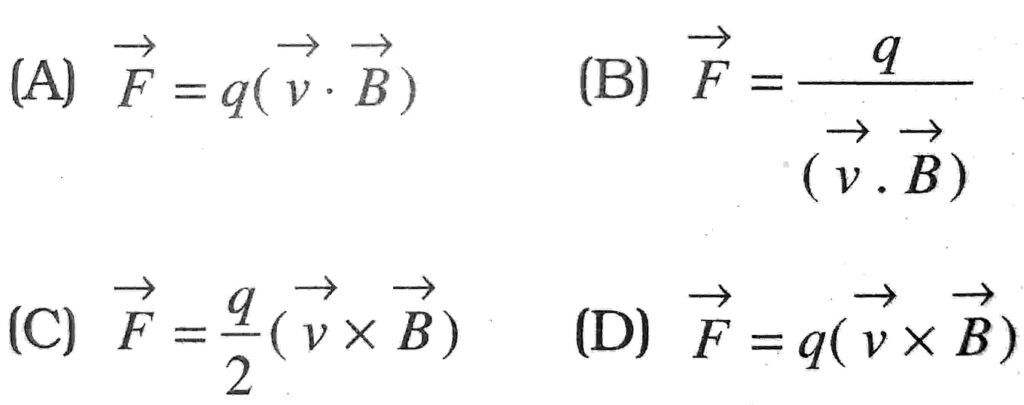16. Which of the following is not a unit of magnetic induction ?

(A) gauss
(B) tesla
(C) oersted
(D) weber/metre2

17. The magnetic field produced by an 1 meter long straight (very thin) current (I) carrying conductor at any point on itself is :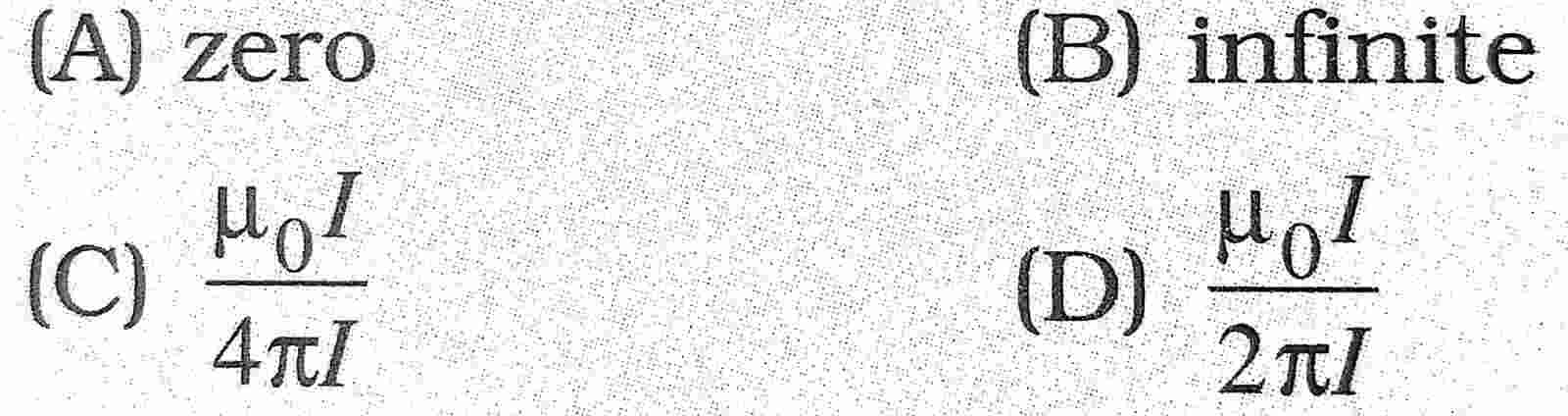18. A charge + q is sent through a magnetic field. The force acting on it is maximum when the angle between the direction of motion of the charged particle and the magnetic field:

(A) 0°
(B) 45°
(C) 90°
(D) 180°

19. An electron having mass ‘m’ and Kinetic energy E enters in uniform magnetic field B perpendicular, then its frequency will be :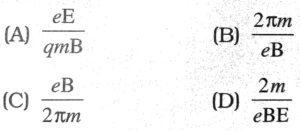20. A wire of length 2 metre carries a current 1 ampere, is bent to form a circle. The magnetic moment of the coil is :

(A) 2π
(B) π/2
(C) π/4
(D) 1/π

21. The magnetic field of a given length of a wire for single turn coil at its centre is B. Then its value for two turns of coil will be :

(A) B/4
(B) B/2
(C) 4B
(D) 2B

22. When charged particle enters a uniform magnetic field, its K.E.

(A) remains constant
(B) increases
(C) decreases
(D) becomes zero

23. To convert galvanometer into voltmeter one should connect :

(A) high resistance in series with galvanometer
(B) low resistance in series with galvanometer
(C) high resistance in parallel with galvanometer
(D) low resistance in parallel with galvanometer

24. The most suitable metal for making permanent magnets is :

(A) iron
(B) steel
(C) copper
(D) aluminium

25. The SI unit of magnetic dipole moment is :

(A) Ampere
(B) Ampere metre2
(C) Tesla
(D) None of these

26. Earth’s magnetism was discovered by :

(A) Gauss
(B) Oersted
(C) Ampere
(D) Gilbert

27. The magnetic moment per unit volume is called :

(A) magnetising field
(B) magnetic induction
(C) magnetisation
(D) magnetic permeability

28. The dimensions of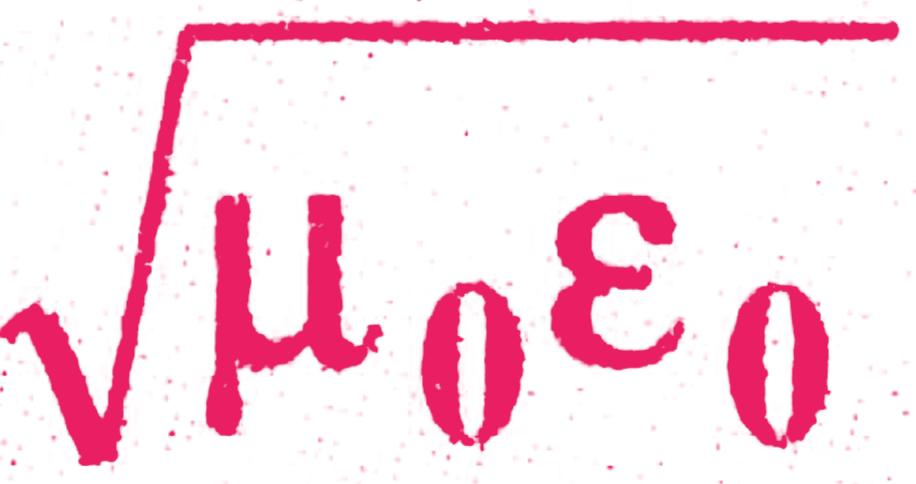are same as that of :

(A) velocity
(B) acceleration
(C) 1/velocity
(D) 1/acceleration

29. The SI unit for μ0 is :

(A) T
(B) TmA-1
(C) TmA-2
(D) Tm2A-1

30. The S.I. unit of magnetic permeability is :

(A) Wb m-2 A-1
(B) Wb m-1 A-1
(C) Wb m-2 A
(D) Wb m A